## TN State Board 11th Computer Science Important Questions Chapter 8 Iteration and Recursion

Question 1.
Define iteration.
In iteration, the loop body is repeatedly executed as long as the loop condition is true. Each time the loop body is executed, the variables are updated. However, there is also a property of the variables which remains unchanged by the execution of the loop body.

Question 2.
Define Recursion.
Recursion is an algorithm design technique, closely related to induction. It is similar to iteration, but more powerful. Using recursion, a problem can be solved with a given input, by solving the instances of the problem with a part of the input.

Question 3.
What is Base case.
The problem size is small enough to be solved directly. Output the solution. There must be at least one base case.Question 4.
Define recursive call.
To solve a problem recursively, the solver reduces the problem to sub-problems and calls another instance of the solver, known as sub-solver, to solve the sub-problem. The input size to a sub-problem is smaller than the input size to the original problem. When the solver calls a sub-solver, it is known as recursive call.

Question 5.
Give the difference between Recursion and Iteration.

 Recursion Iteration The statement in a body of function calls the function itself. Allows the set of instructions to be executed repeatedly. It is always applied to functions. It is applied to iteration statements or “loops”. It reduces the size of the code. It makes the code longer.

Question 6.
If we execute the following assignment with, (p, c = 10, 9) after the assignment (u, v) = (11, 10) discover an invariant. What is the value of p – c before and after?
— before : p, c = 10, 9
p, c := p + 1, c + 1
— after: p, c = 11, 10
before: p – c = 10 – 9 = 1
after: p – c = 11 – 10 = 1
We find that p – c = 1 is an invariant.Question 7.
Show that p – c is an invariant of the assignment.
p, c := p + 1, c + 1
Let P(p, c) = p – c. Then
P (p, c) [p, c := p + 1, c + 1]
= p – c [p, c := p + 1, c + 1]
= (p + 1) – (c + 1)
= p – c
= p(P, c)
Since (p – c) [p, c := p + 1, c + 1] = p – c, p – c is an invariant of the assignment p, c := p + 1, c+ 1.

Question 8.
There are 6 equally spaced trees and 6 sparrows sitting on these trees, one sparrow on each tree. If a sparrow flies from one tree to another, then at the same time another sparrow flies from its tree to some other tree the same distance away, but in the opposite direction. It is possible for all the sparrows to gather on one tree?
Let us index the trees from 1 to 6. The index of a sparrow is, the index of the tree it is currently sitting on. A pair of sparrows flying can be modeled as an iterative step of a loop. When a sparrow at tree i flies to tree i + d, another sparrow at tree j flies to tree j – d. Thus, after each iterative step, the sum S of the indices of the sparrows remains invariant. Moreover, a loop invariant is true at the start and at the end of the loop.

At the start of the loop, the value of the invariant is or White White. It is illustrated in Figure and annotated in the algorithm below.

S = 1 + 2 + 3 + 4 + 5 + 6 = 21

When the loop ends, the loop invariant has the same value. However, when the loop ends, if all the sparrows were on the same tree, say k, then S = 6k.

 S = 21, loop invariant at the start of the loop S = 6k, loop invariant at end of the loop 6k = 21, loop invariant has the same value at the start and the end 21 is a multiple of 6

It is not possible. 21 is not a multiple of 6. The desired final values of the sparrow indices is not possible with the loop invariant. Therefore, all the sparrows cannot gather on one tree.Question 9.
You are given a jar full of two kinds of marbles, white and black, and asked to play this game. Randomly select two marbles from the jar. If they are the same color, throw them out, but put another black marble in (you may assume that you have an endless supply of spare marbles). If they are different colors, place the white one back into the jar and throw the black one away. If you knew the original numbers of white and black marbles, what is the color of the last marble in the jar?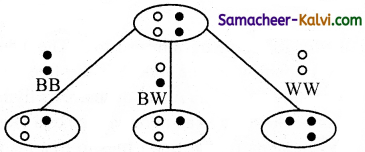The number of white and black marbles in the jar can be represented by two variables w and b. In each iterative step, b and w change depending on the colors of the two marbles taken out: Black Black, Black White or White White. It is illustrated in Figure and annotated in the algorithm below.

1 while at least two marbles in jar
2 b, w
3 take out any two marbles
4 case both are black– BB
5 throw away both the marbles
6 put a black marble back
7 — b = b’1-, w = w’, b+w = b’+w’1- 8 case both are white-W W
9 throw away both the marbles
10 put a black marble back
11 b = b’1+, w = w’2-, b+w = b’+w’1-
12 else –BW
13 throw away the black one
14 put the white one back
15 — b = b’1-, w = w’, b+w = b’+w’1-

For each case, how b, w and b+w change is shown in the algorithm, where b’ and w’ are values of the variables before taking out two marbles. Notice the way w changes. Either it does not change, or decreases by 2. This means that the parity of w, whether it is odd or even, does not change. The parity of w is invariant.

Suppose, at the start of the game, w is even. When the games ends, w is still even. Moreover, only one marble is left, w + b = 1.

 1 w + b = 1 end of the loop 2 w = 0 or w = 1 from 1 3 w is even loop invariant 4 w = 0 from 2, 3 5 b = 1 from 1, 4

Last marble must be black. Similarly, if at the start of the game, there is an odd number of whites, the last marble must be white.Question 10.
Explain in detail the Recursion problem solving technique.
To solve a problem recursively, the solver reduces the problem to sub-problems, and calls another instance of the solver, known as sub-solver, to solve the sub-problem. The input size to a sub-problem is smaller than the input size to the original problem. When the solver calls a sub-solver, it is known as recursive call.

The magic of recursion allows the solver to assume that the sub¬solver (recursive call) outputs the solution to the sub-problem. Then, from the solution to the sub-problem, the solver constructs the solution to the given problem.

As the sub-solvers go on reducing the problem into sub-problems of smaller sizes, eventually the sub-problem becomes small enough to be solved directly, without recursion. Therefore, a recursive solver has two cases:
(i) Base case:
The problem size is small enough to be solved directly. Output the solution. There must be at least one base case.

(ii) Recursion step:
The problem size is not small enough. Deconstruct the problem into a sub-problem, strictly smaller in size than the given problem. Call a sub solver to solve the sub-problem. Assume that the sub-solver outputs the solution to the sub-problem. Construct the solution to the given problem.
This outline of recursive problem solving technique is shown below.
solver (input)
if input is small enough
construct solution
else
find sub_problems of reduced input solutions to sub_problems = solver
for each sub_problem construct solution to the problem from solutions to the sub_problems

Whenever a problem is solved using recursion, these two cases have to be ensured. In the recursion step, the size of the input to the recursive call is strictly smaller than the size of the given input and there is at least one base case.

Question 11.
What is an invariant?
An invariant is a condition that can be relied upon to be true during execution of a program or during some portion of it. It is a logical assertion that is held to always be true during a certain phase of execution.Question 12.
Define a loop invariant.
A loop invariant is a condition that is necessarily true immediately before and immediately after each iteration of a loop.

Question 13.
Does testing the loop condition affect the loop invariant? Why?
No. It does not affect the loop invariant.

1. at the start of the loop (just before the loop)
2. at the start of each iteration (before loop body)
3. at the end of each iteration (after loop body)
4. at the end of the loop (just after the loop)

Question 14.
What is the relationship between loop invariant, loop condition and the input – output recursively?
(i) Establish the loop invariant at the start of the loop.
(ii) The loop body should so update the variables as to progress toward the end and maintain the loop invariant, at the same time.
(iii) When the loop ends, the termination condition and the loop invariant should establish the input-output relation.Question 15.
What is recursive problem solving?
Each solver should test the size of the input. If the size is small enough, the solver should output the solution to the problem directly. If the size is not small enough, the solver should reduce the size of the input and call a sub-solver to solve the problem with the reduced input.

Question 16.
Define factorial of a natural number recursively.
The factorial of a natural number defined recursively as the base case can be taken as the factorial of the number 0 or 1, both of which are 1. The factorial of some number n is that number multiplied by the factorial of (n -1).

Question 17.
There are 7 tumblers on a table, all standing upside down. You are allowed to turn any 2 tumblers simultaneously in one move. Is it possible to reach a situation when all the tumblers are right side up? (Hint: The parity ‘of the number of upside down tumblers is invariant.)Answer:
(i) u is the number of tumblers upside down 3 cases.
(ii) 1 turn two tumblers the right way up (w: = u + 2)
(iii) 2 turn two tumblers the wrong way up (u: = u- 2)
(iv) 3 turn one the right way up and the other the wrong way up (u: = u + 1 – 1)
The invariant of these assignments.
(i) Parity is a Boolean value (true or false)
(ii) True if (0, 2, 4, 6 ….)
(iii) False if (1, 3, 5, 7….)
(iv) Notation even u
(v) Invariant of u: – u + 2
(vi) Invariant of u: = u – 2
(vii) Even u is an invariant of the problem-
(viii) No matter how many times we turn over pairs of tumbler, the value is even. So It is not possible to reach the situation when all the tumblers are right side up.Question 18.
A knockout tournament is a series of games. Two players compete in each game; the loser is knocked out (i.e. does not play any more), the winner carries on. The winner of the tournament is the player that is left after all other players have been knocked out. Suppose there are 1234 players in a tournament. How many games are played before the tournament winner is decided?
Let p be no. of players
Let g be no. of games
initially p = 1234, g = 0
p, g: = p-l,g + l
p + g is invariant
finally p = 1, g = 1233.

Question 19.
King Vikramaditya has two magic swords. With one, he can cut off 19 heads of a dragon, but after that the dragon grows 13 heads. With the other sword, he can cut off 7 heads, but 22 new heads grow. If all heads are cut off, the dragon dies. If the dragon has originally 1000 heads, can it ever die? (Hint:The number of heads mod 3 is invariant.)
So (19 -13) = 6 = 0 (mod 3)
So (22 – 7) = 15 = 0 (mod 3)
We note (19 – 13) = (22 – 7) = 0 (mod 3)
The magic swords can never change the number of heads of the dragon mod 3.
Since we start at 1000 = 1 (mod 3) we can never get to 0. The dragon lives.Question 20.
Assume an 8 × 8 chessboard with the usual coloring. “Recoloring” operation changes the color of all squares of a row or a column. You can recolor re-peatedly. The goal is to attain just one black square. Show that you cannot achieve the goal. (Hint: If a row or column has b black squares, it changes by (|8 – b) – b|).
We start with a normal coloured chess board with number of black squares B = 32 and number of white squares W = 32.
So W – B = 0 which is divisible by 4 and W + B = 64 W – B = 0 mod 4.
Whenever we change the colours of a row or column, we change the colour of 8 squares. Let this row (or column) have W white squares + b black squares w + b = 8 squares. If this operation B increases by 2n, then W decreases by 2n so that W + B = 64 but B – W will change by 4n and if will remain divisible by 4.
W – B = 0 mod 4.
After every operation ‘B – W mod 4” can have no other values.
But the required state has 63 white squares and 1 black square, so it requires.
W – B = 63 – 1 = 62 = 2 mod 4. which is impossible.

Question 21.
Power can also be defined recursively as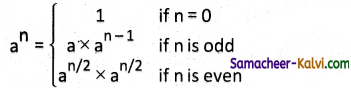Construct a recursive algorithm using this definition. How many multiplications are needed to calculate a 10?
power (a, n)
— inputs : n is an integer; n > 0
–outputs: an
if n = 0–base case
1
else if n is odd then
a × power (a, n – 1)
else
power (a, n/2) × power (a, n/2)Question 22.
A single-square-covered board is a board of 2n × 2n squares in which one square is covered with a single square tile. Show that it is possible to cover this board with triominoes without overlap.
The size of the problem is n (board of size 2n × 2n). We can solve the problem by recursion. The base case is n = 1. It is a 2 × 2 single-covered board. We can cover it with one triominoe and solve the problem. In the recursion step, divide the single-covered board of size 2n × 2n into 4 sub-boards, each of size 2n – 1 × 2n – 1, by drawing horizontal and vertical lines through the centre of the board. Place atriominoe at the centre of the entire board so as to not cover the single- covered sub-board, as shown in the left-most board of figure. Now, we have four single-covered boards, each of size 2n – 1 × 2n – 1.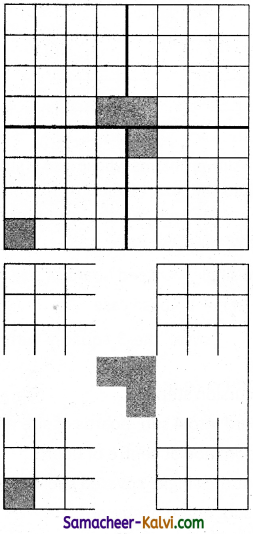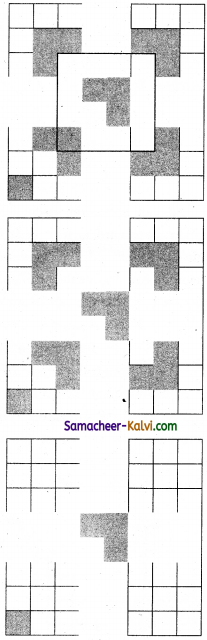Question 1.
In _________ the loop body is repeatedly executed as long as the loop condition is true.
(a) iteration
(b) recursion
(c) function
(d) statement
(a) iteration

Question 2.
________ is a method call to the same method.
(a) Algorithm
(b) Recursion
(c) Function
(d) Loop
(b) Recursion

Question 3.
Who coined the phrase “structured programming”?
(a) Charles Babbage
(b) Douglas Engelbart
(c) George Boole
(d) Dijkstra
(d) DijkstraQuestion 4.
Suppose u, v = 20, 15 before the assignment what are the values of u and v after the assignment statement, u, v := u + 5, v – 5
(a) u, v = 10, 25
(b) u, v = 20, 10
(c) u, v = 25, 10
(d) u, v = 25, 5
(c) u, v = 25, 10

Question 5.
The loop invariant is true in ______ crucial points in a loop.
(a) two
(b) three
(c) four
(d) five
(c) four

Question 6.
There must be atleast _____ base case.
(a) one
(b) two
(c) three
(d) four
(a) oneQuestion 7.
Who was one of the most influential pioneers of computing science?
(a) E.W. Dijkstra
(b) Douglas Engelbart
(c) George Boole
(d) G Polya
(a) E.W. Dijkstra

Question 8.
A loop invariant need not be true:
(a) at the start of the loop.
(b) at the start of each iteration
(c) at the end of each iteration
(d) at the start of the algorithm
(d) at the start of the algorithm

Question 9.
We wish to cover a chessboard with dominoes, the number of black squares and the number of white squares covered by dominoes, respectively, placing a domino can be modeled by:
(a) b := b + 2
(b) w := w + 2
(c) b, w := bl+, wl+
(d) b:= w
(c) b, w := bl+, wl+Question 10.
If m × a + n × b is an invariant for the assignment a, b : = a + 8, b + 7, the values of m and n are:
(a) m = 8, n = 7
(b) m = 7, n = – 8
(c) m = 7, n = 8
(d) m = 8, n = -7
(b) m = 7, n = – 8

Question 11.
Which of the following is not an invariant of the assignment?
m, n := m+2, n+3
(a) m mod 2
(b) n mod 3
(c) 3 × m – 2 × n
(d) 2 × m – 3 × n
(c) 3 × m – 2 × n

Question 12.
If Fibonacci number is defined recursively as: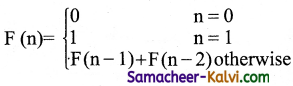to evaluate F(4), how many times F( ) is applied?
(a) 3
(b) 4
(c) 8
(d) 9
(b) 4Question 13.
Using this recursive definition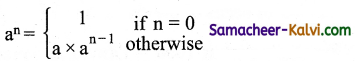how many multiplications are needed to calculate a10?
(a) 11
(b) 10
(c) 9
(d) 8